### Discover more content...

Enter some keywords in the search box above, we will do our best to offer you relevant results.

### We're sorry!

We couldn't find any results for your search. Please try again with another keywords.

## 一. Gradient Descent with Large Datasets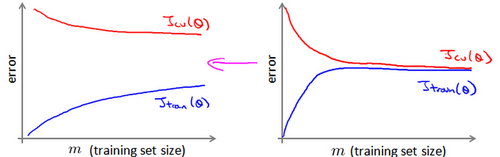$$\theta_j=\theta_j-\alpha \frac{1}{m} \sum_{i=1}^{m}(h_{\theta}(x^{(i)})-y^{(i)})x^{(i)}_j,\;\;\;\;for\;\;j=0,\cdots,n$$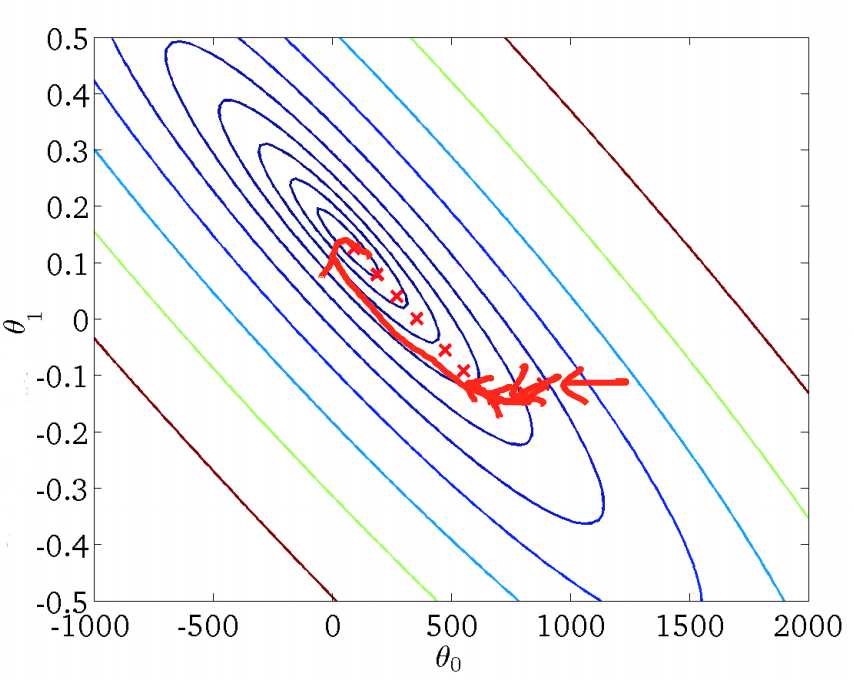\begin{aligned} for \;\; i&=1,\cdots,m:\\ \theta_j&=\theta_j-\alpha(h_\theta(x^{(i)})-y^{(i)})x^{(i)}_j, \;\; for \;\;j=0,\cdots,n\\ \end{aligned}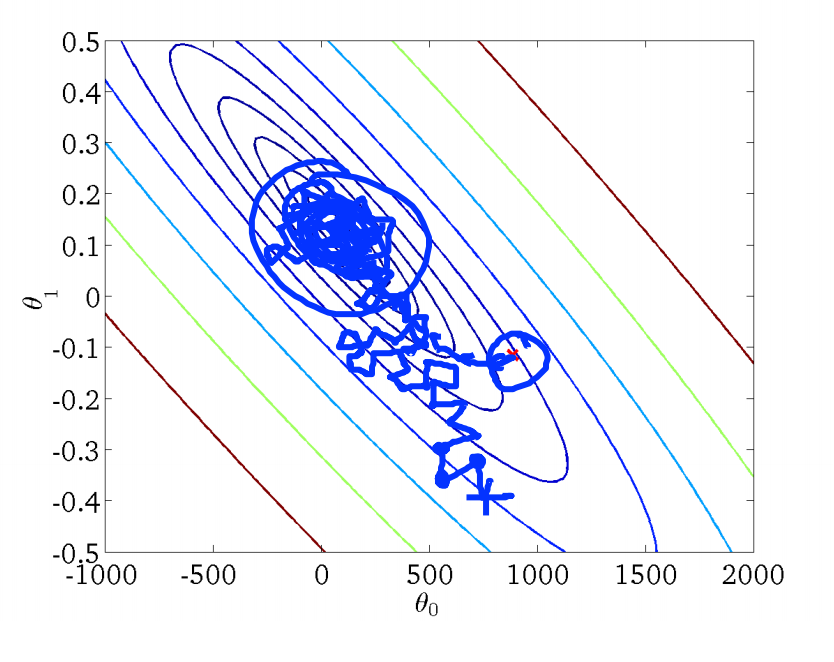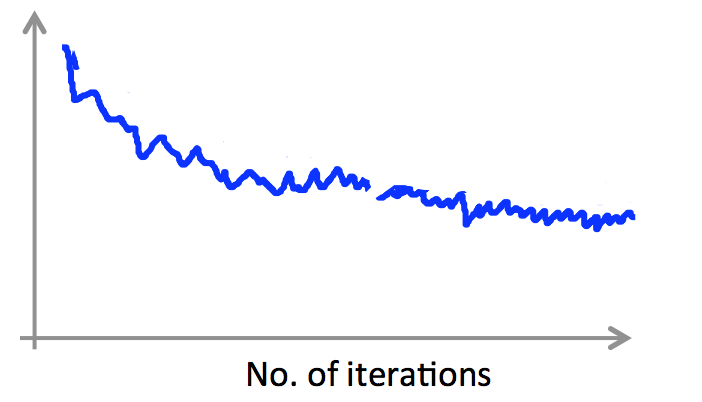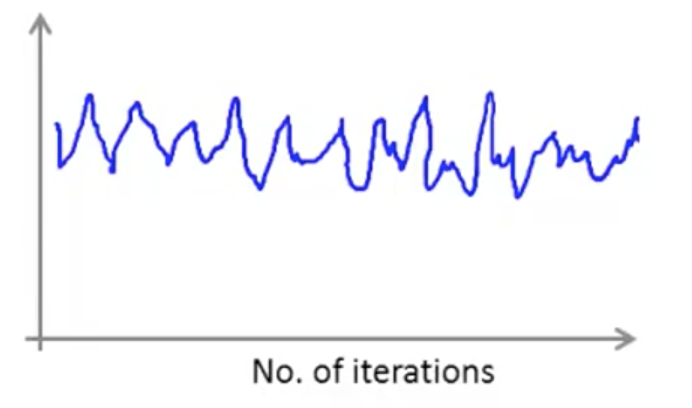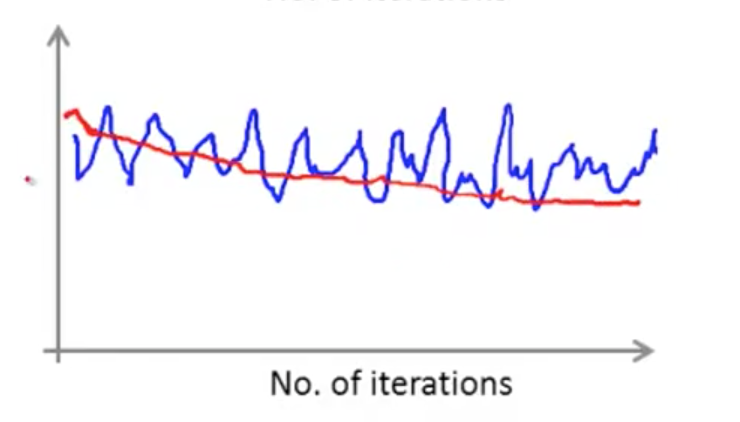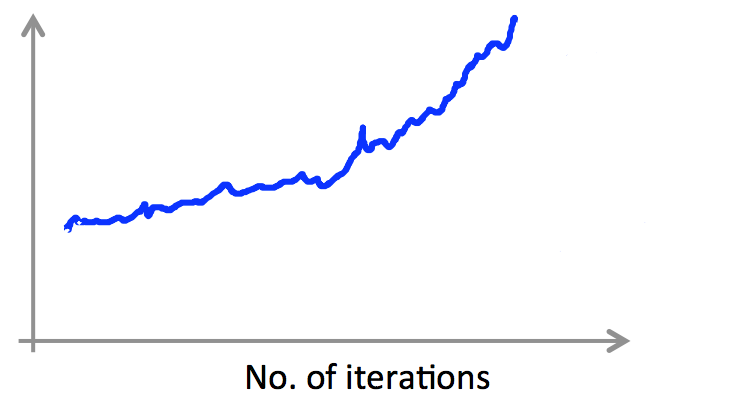$$\alpha=\frac{constant1}{iterationNumber+constant2}$$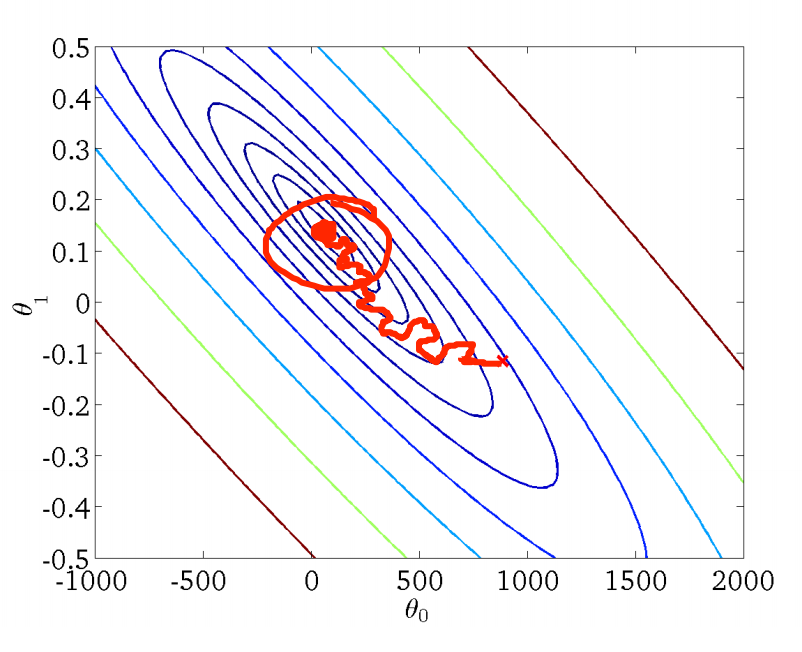### 3. Mini 批量梯度下降法（Mini-batch gradient descent）

Mini 批量梯度下降法是批量梯度下降法和随机梯度下降法的折中，通过参数 b 指明了每次迭代时，用于更新 $\theta$ 的样本数。假定 b=10,m=1000 ，Mini 批量梯度下降法的工作过程如下：

\begin{align*} for\;\;\;i&=1,11,21,\cdots,991:\\ \theta_j&=\theta_j-\alpha \frac{1}{10}\sum_{k=i}^{i+9}(h_\theta(x^{(i)})-y^{(i)})x^{(i)}_j,\;\;\;\;for\;\;j=0,\cdots,n\\ \end{align*}

### 4. 在线学习

$$\theta_j=\theta_j-\alpha(h_\theta(x)-y)x_j,\;\;\;for\;\;j=0,\cdots,n$$

### 5. MapReduce

$$\theta_j=\theta_j-\alpha \frac{1}{400} \sum i=1^{400}(h_\theta(x^i)-y^{(i)})x^{(i)}_j$$

\begin{align*} temp^{(1)}_j&=\sum_{i=1}^{100}(h_\theta(x^{(i)}-y^{(i)})x^{(i)}_j\\ temp^{(2)}_j&=\sum_{i=101}^{200}(h_\theta(x^{(i)}-y^{(i)})x^{(i)}_j\\ temp^{(3)}_j&=\sum_{i=201}^{300}(h_\theta(x^{(i)}-y^{(i)})x^{(i)}_j\\ temp^{(4)}_j&=\sum_{i=301}^{400}(h_\theta(x^{(i)}-y^{(i)})x^{(i)}_j\\ \end{align*}

$$\theta_j=\theta_j-\alpha \frac{1}{400}(temp_j^{(1)}+temp_j^{(2)}+temp_j^{(3)}+temp_j^{(4)})$$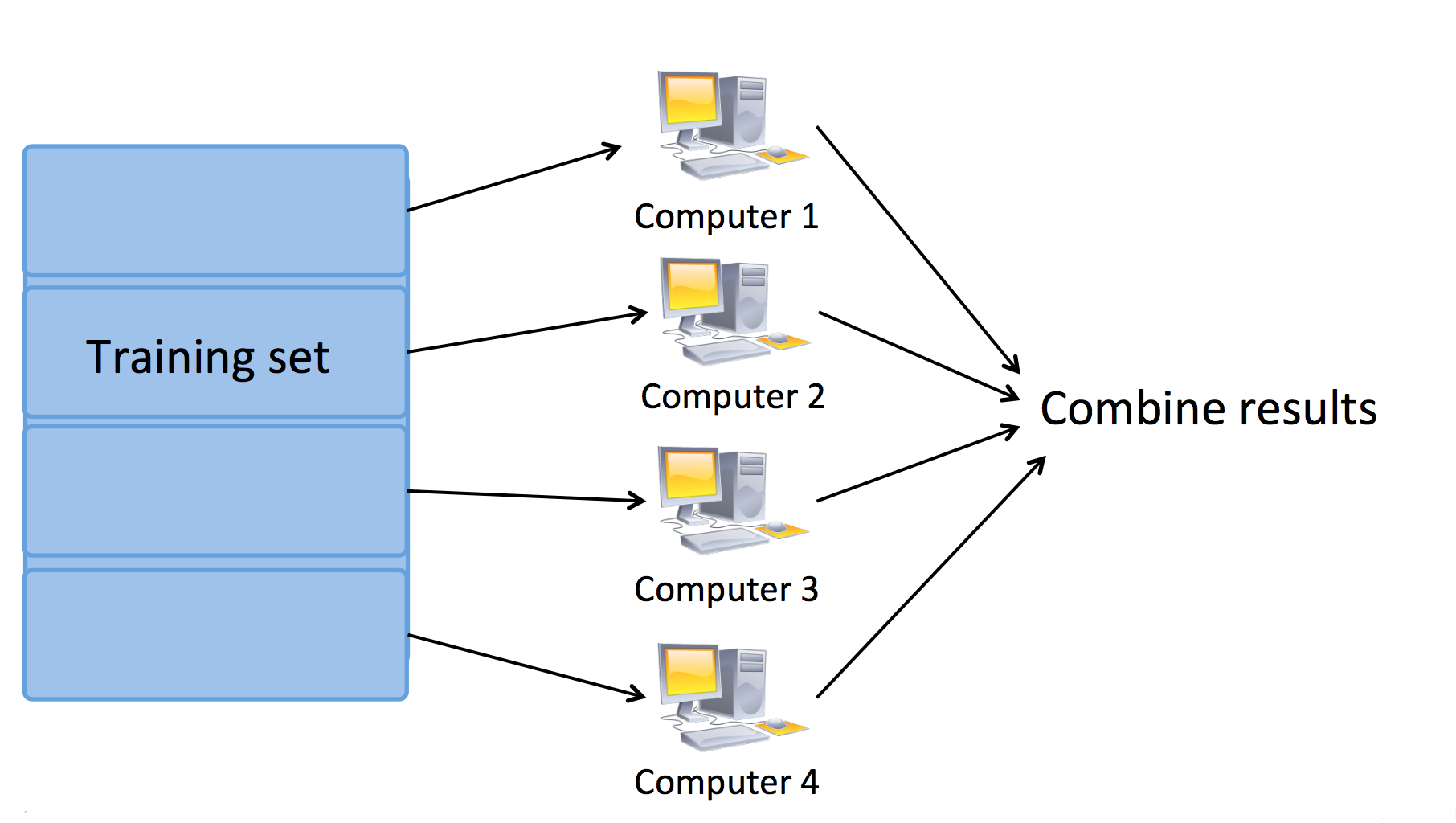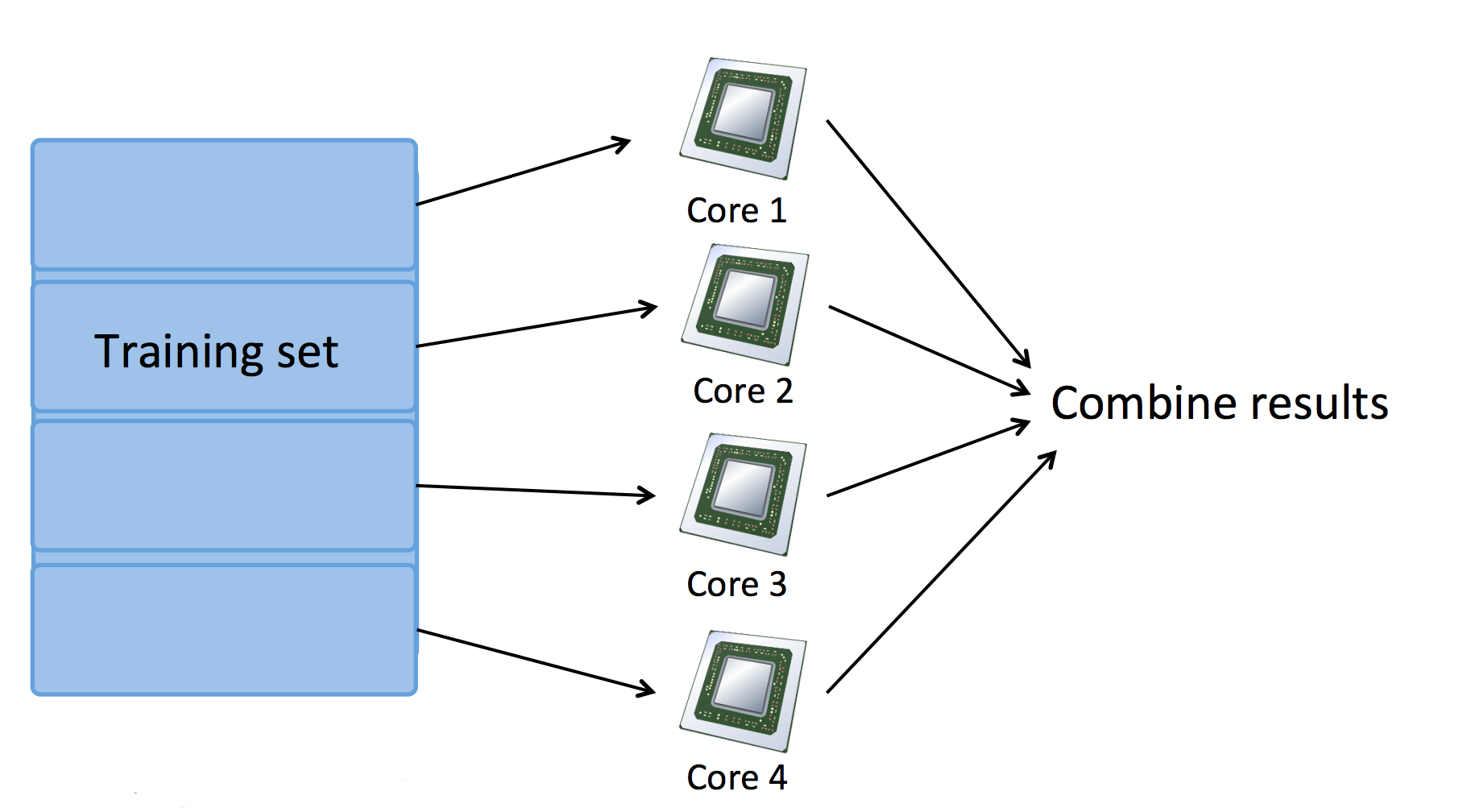## 三. Large Scale Machine Learning 测试

### 1. Question 1

Suppose you are training a logistic regression classifier using stochastic gradient descent. You find that the cost (say, $cost(\theta,(x^{(i)},y^{(i)})$), averaged over the last 500 examples), plotted as a function of the number of iterations, is slowly increasing over time. Which of the following changes are likely to help?

A. Use fewer examples from your training set.

B. Try averaging the cost over a smaller number of examples (say 250 examples instead of 500) in the plot.

C. This is not possible with stochastic gradient descent, as it is guaranteed to converge to the optimal parameters $\theta$.

D. Try halving (decreasing) the learning rate $\alpha$, and see if that causes the cost to now consistently go down; and if not, keep halving it until it does.

### 2. Question 2

Which of the following statements about stochastic gradient descent are true? Check all that apply.

A. Stochastic gradient descent is particularly well suited to problems with small training set sizes; in these problems, stochastic gradient descent is often preferred to batch gradient descent.

B. In each iteration of stochastic gradient descent, the algorithm needs to examine/use only one training example.

C. Suppose you are using stochastic gradient descent to train a linear regression classifier. The cost function $J(\theta)=\frac{1}{2m}\sum^m_{i=1}(h_\theta(x^{(i)})-y^{(i)})^2$ is guaranteed to decrease after every iteration of the stochastic gradient descent algorithm.

D. One of the advantages of stochastic gradient descent is that it can start progress in improving the parameters $\theta$ after looking at just a single training example; in contrast, batch gradient descent needs to take a pass over the entire training set before it starts to make progress in improving the parameters' values.

E. n order to make sure stochastic gradient descent is converging, we typically compute $J_{train}(\theta)$ after each iteration (and plot it) in order to make sure that the cost function is generally decreasing.

F. If you have a huge training set, then stochastic gradient descent may be much faster than batch gradient descent.

G. In order to make sure stochastic gradient descent is converging, we typically compute $J_{train}(\theta)$ after each iteration (and plot it) in order to make sure that the cost function is generally decreasing.

H. Before running stochastic gradient descent, you should randomly shuffle (reorder) the training set.

C 错误
G 并不需要代价函数总是减少，可能会降低故错误

### 3. Question 3

Which of the following statements about online learning are true? Check all that apply.

A. Online learning algorithms are most appropriate when we have a fixed training set of size m that we want to train on.

B. Online learning algorithms are usually best suited to problems were we have a continuous/non-stop stream of data that we want to learn from.

C. When using online learning, you must save every new training example you get, as you will need to reuse past examples to re-train the model even after you get new training examples in the future.

D. One of the advantages of online learning is that if the function we're modeling changes over time (such as if we are modeling the probability of users clicking on different URLs, and user tastes/preferences are changing over time), the online learning algorithm will automatically adapt to these changes.

### 4. Question 4

Assuming that you have a very large training set, which of the following algorithms do you think can be parallelized using map-reduce and splitting the training set across different machines? Check all that apply.

A. Logistic regression trained using batch gradient descent.

B. Linear regression trained using stochastic gradient descent.

C. Logistic regression trained using stochastic gradient descent.

D. Computing the average of all the features in your training set $\mu=\frac{1}{m}\sum^m_{i=1}x^{(i)}$ (say in order to perform mean normalization).

B. Linear regression trained using batch gradient descent. 所以 B 错误

C. 错误

### 5. Question 5

Which of the following statements about map-reduce are true? Check all that apply.

A. When using map-reduce with gradient descent, we usually use a single machine that accumulates the gradients from each of the map-reduce machines, in order to compute the parameter update for that iteration.

B. Linear regression and logistic regression can be parallelized using map-reduce, but not neural network training.

C. Because of network latency and other overhead associated with map-reduce, if we run map-reduce using N computers, we might get less than an N-fold speedup compared to using 1 computer.

D. If you have only 1 computer with 1 computing core, then map-reduce is unlikely to help.

E. If you have just 1 computer, but your computer has multiple CPUs or multiple cores, then map-reduce might be a viable way to parallelize your learning algorithm.

F. In order to parallelize a learning algorithm using map-reduce, the first step is to figure out how to express the main work done by the algorithm as computing sums of functions of training examples.

G. Running map-reduce over N computers requires that we split the training set into $N^2$ pieces.

B 错误
C 正确
E 错误
F 错误
G 可能正确？

GitHub Repo：Halfrost-Field

Follow: halfrost · GitHubPrevious Post

Next Post